[BACK]
 Computers, Materials & ContinuaDOI:10.32604/cmc.2022.019921Article

FPGA Implementation of Deep Leaning Model for Video Analytics

Mahendra College of Engineering, Salem, India
*Corresponding Author: P. N. Palanisamy. Email: pnpalanisamyece@gmail.com
Received: 01 May 2021; Accepted: 12 July 2021

Abstract: In recent years, deep neural networks have become a fascinating and influential research subject, and they play a critical role in video processing and analytics. Since, video analytics are predominantly hardware centric, exploration of implementing the deep neural networks in the hardware needs its brighter light of research. However, the computational complexity and resource constraints of deep neural networks are increasing exponentially by time. Convolutional neural networks are one of the most popular deep learning architecture especially for image classification and video analytics. But these algorithms need an efficient implement strategy for incorporating more real time computations in terms of handling the videos in the hardware. Field programmable Gate arrays (FPGA) is thought to be more advantageous in implementing the convolutional neural networks when compared to Graphics Processing Unit (GPU) in terms of energy efficient and low computational complexity. But still, an intelligent architecture is required for implementing the CNN in FPGA for processing the videos. This paper introduces a modern high-performance, energy-efficient Bat Pruned Ensembled Convolutional networks (BPEC-CNN) for processing the video in the hardware. The system integrates the Bat Evolutionary Pruned layers for CNN and implements the new shared Distributed Filtering Structures (DFS) for handing the filter layers in CNN with pipelined data-path in FPGA. In addition, the proposed system adopts the hardware-software co-design methodology for an energy efficiency and less computational complexity. The extensive experimentations are carried out using CASIA video datasets with ARTIX-7 FPGA boards (number) and various algorithms centric parameters such as accuracy, sensitivity, specificity and architecture centric parameters such as the power, area and throughput are analyzed. These results are then compared with the existing pruned CNN architectures such as CNN-Prunner in which the proposed architecture has been shown 25% better performance than the existing architectures.

Keywords: Deep neural networks; field programmable gate arrays;; convolutional neural networks; distributed filtering structures; bat-pruned

1  Introduction

Deep neural networks (DNN) in recent years has achieved the remarkable growth and considered to be the powerful candidate for a variety of applications such as Image processing, video analytics and speech processing .

Currently, Convolutional Neural Networks (CNN) has gained its new lime light for handling the video processing. Because of its hardware versatility and ability to provide high performance per unit power, FPGA is becoming an integral part of implementing CNN accelerators . The Graphics Processing Unit (GPU), on the other hand, provides us with more memory and computing power . Because of its hardware versatility and ability to provide high performance per unit power, FPGA is becoming an integral part of implementing CNN accelerators . Owing to the small number of hardware resources, FPGA also has limitations.

Several Methods such as hard thresholds methods, Automatic Filtering Methods, Block Pruning methods were assumed to reach the saturation in compression without sacrificing the model's accuracy to make it more suitable for the FPGA architectures . Furthermore, the complex interplay between the stages of filter pruning and model fine-tuning makes this process difficult to control . As a result, current pruned accelerators are unable to optimally balance the trade-off between pruning efficiency and hardware resources . Focused on the above challenges, the paper proposes the new hybrid evolutionary Bat pruned deep hybrid convolutional neural network models to establish the good tradeoff between the pruning efficiency, prediction accuracy and the hardware usage. Since the pruning of the network may have adversely impact on the video frames, the paper incorporates the effective selection of weights to be removed and boosted LSTM to maintain the good accuracy in prediction. The contribution of the paper is as follows

1)

The paper proposed new Evolutionary Pruned Hybrid Convolutional Neural Networks for processing the input video sequences. The hybrid convolutional networks have been formulated with the replacement of fully connected neural network layers with the boosted Long short term memory layers for the better prediction. To select the accurate removal of weights, Bat algorithm has been incorporated to maintain the good pruning efficiency which may have less impact on video sequences.

2)

Incorporation of the Distributed Filtering Structures for the implementing the proposed architecture to manage the resources in FPGA to achieve the energy efficient and high performance.

3)

The introduction of Hardware -Software Codesign for an effective implementation of the models with the more flexible in terms of user defined mechanism.

The manuscript is arranged as per: Section 2 describes the background and relevant works by the different authors in pruning techniques and hybrid learning models for videos sequences. The preliminary overview of convolutional neural network, Long short term memory, Bat Algorithms has been presented in the Section-3. The proposed architecture's working process and FPGA implementation of the proposed model are discussed in Section 5, and the results are presented in Section 6. Finally, section 7 brings the paper to a close.

2  Related Works

Xu et al.  proposed an idea to measure the visual quality based on supervised machine learning model. For this, a synthetic dataset that contains low-light and various degrees of haze are produced. A Multivariate Statistic Gaussian model (MVG) is then trained with a particular mixture for each degree of exposure of the global content. The blurry and hazy images used to train the visibility estimation model are composite images of the ground truth data. The enhancement model deployed can exhibit poor visual quality if the bin number decreases.

Chen et al.  developed a pipeline model for depth estimation in stereo applications. To learn a differential binary descriptor, the hardware-friendly operation with binary neural network (BNN) is used. The Stereo Engine is a standalone specification for a DNN-based stereo viewing device that implements all processing procedures on the hardware platform. Stereo Engine provides a high-level pipeline between accelerators and image sensors without an external processor or GPU. Integration of optimization layer and repetitive convolution are performed to enhance the hardware efficiency. The matching accuracy need to be improved for any hardware end-to-end DNN-based stereo vision accelerator in FPGAs based stereo research real time.

Kim et al.  introduced an efficient multiscale SR hardware based on CNN that can convert 4 K UHD amplifiers to 60 fps. The multi-scale SR tool uses a limited number of filter parameters and does not use a frame buffer to store intermediate feature maps. To further reduce the computational complexity and memory consumption, they used a simple and effective quantization scheme for weights and activations. As a result, the SR HW is capable of reproducing 4 K UHD video at 60 frames per second while also handling multi-scale conditions of 2, 3, and 4.

Yang et al.  developed an implementation for the evaluation in sports field to judge claims of opponents using image verification. CNN and FPGA support have been involved in identifying images of sports activities. The number of CNN layers and the purpose of the information loop have been reduced to meet limited memory requirements. As a result, the unchanged image is correctly classified as one of the amount of data involved, and FPGA acceleration reduces the transfer time. But a subjective study of the model often leads to non-predictable errors due to the environmental changes.

Cosmas et al.  proposed an energy-efficient and cost-effective on-board interfacing for spacecraft pose estimation. The proposed scheme uses a FPGA and system on a chip (SoC) device for CNN capture in these gesture estimation techniques. This study uses a Xilinx ZynqUltraScale + MPSoC device, which is provided as an efficient acquisition solution on board. This work needs further improvement to ensure that the spacecraft is in FPGA-based domain from the start to finish. This includes the interaction between the real-time camera and CPU-based algorithms for real time deployment.

Han et al.  adopted CNN with hardware-friendly advanced gateways. Word performance and network depth were further extended to improve model performance. Sigmoid functions were replaced to make it more convenient for lossless hardware computing. The FPGA based event detection accelerator has greatly reduced latency through its complete pipeline architecture. The Xilinx XCKU115 FPGA is used to implement the accelerator. The model was found to have the highest F1 score of 84.6 percent in the ACE kit. For real-time applications, the model fails to connect event detection and event argument extraction.

Yu et al.  introduced a single FPGA chip-based eye tracking device. Our experimental results showed an apparent recognition rate of 0.52 μs and an average detection rate of 92% at a 100 MHz system clock. The use of DSP and memory chips is intentionally increased in the hardware-based implementation, but the detection rate is lower than in software-based versions.

Zhang et al.  presented a VHDL/HLS description of an FPGA pipeline design that can capture events from a DVS retina with an Incident Reference (AER) to generate a normalized histogram that can be used by a dedicated CNN accelerator called NullHop. The measured acceleration rate of 67% is for CNN's Roshambo real-time experiment running at a top speed of 160 frames per second

3  Preliminary Overview

This section discusses about the preliminary overview of the different models such as convolutional neural networks, long short term memory and Bat algorithms.

3.1 Convolutional Neural Networks

Convolutional Neural Network is the popular model among other deep learning models, which finds its applications in image processing and video analytics. The structure of the traditional CNN is shown in Fig. 1.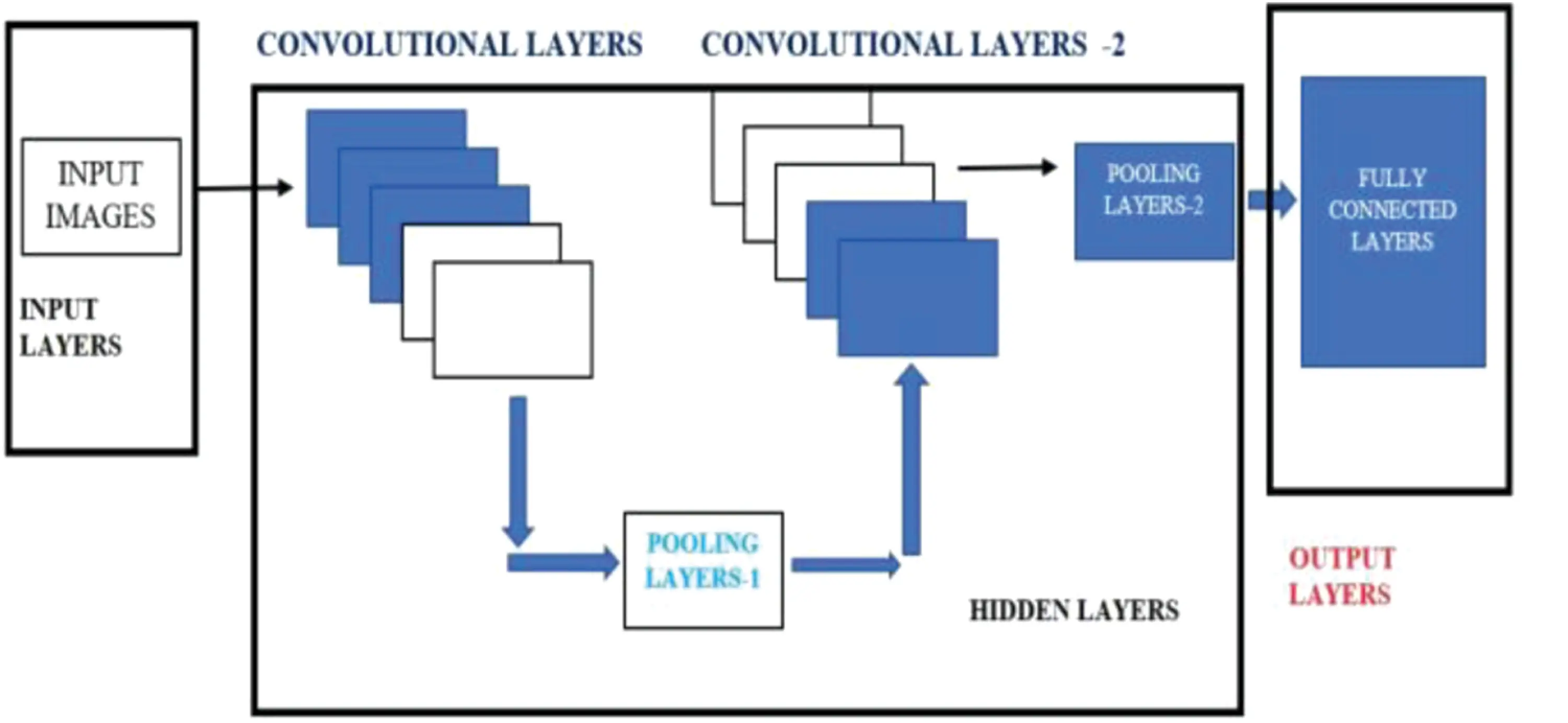Figure 1: Block diagram for the traditional convolutional neural networks

The standard CNN model comprises of three significant operations such as product, filtering, and pooling at the initial stage. The input layer is scrutinized by convolutional operation and feed-forwarded into pooling layer for filtering process. These two steps are mandatory in CNN in order to minimize the output complexities and over-fitting problem. A CNN's fully linked (FC) or dense layers link all neurons from previous layers, allowing for global correlation of complex features derived from product layers. A number of kernels are present in each completely connected layer. These kernels, on the other hand, are only added to the input map once in dense layers. Dense layers, as a result, are not computationally efficient. Instead, the large number of weights to be moved from external memory is the bottleneck of dense layers. Standard CNNs are those that have these three types of layers. AlexNet and VGG16 are two examples of standard networks. In their CNNs, some ideas consider other forms of layers, such as convolutional layer combinations. Networks such as GoogleNet  and ResNet  are referred to as irregular networks because they contain composite layers that differ from the three discussed above .

3.2 LSTM – Long Short Term Memory

A LSTM network is the type of reinforcement learning model, which is primarily used for sequence prediction problems. Cell, input gate, output gate, and forget gate make up this network. To recall values over time periods, cells are considered to be LSTM memories. The cell input state is Ct, the cell output state is Gt, and its former state is Gt-1, and the three gates’ states are T0. Both Gt and ht are transmitted to the next neural network in RNN, according to the structure of the LSTM cell. The output and forget gates are used to change the memory in the LSTM, which combines the output of the previous unit with the current input state. To calculate Gt and ht, in order to do so, we use the equations below. To begin, determine the states of the three gates as well as the cell input state, input gate: The input gate is given as

jt=θ(GliOt+Ghiet1+si)(1)

The forget gate is given as

Tf=θ(GlfOt+Ghfet1+sf)(2)

Output gate is calculated as

To=θ(Gl0Ot+Ghoet1+so)(3)

Cell Input is given as

TC~=tanh(GlCOt+GhCet1+sC)(4)

where Gl0, Glf,Gli,GlC are the weight matrices connecting the input gates to the target layers whereas Ghi,Ghf, Gho,GhC are the weight matrices connecting the gate inputs to the concealed layers. Also si, sf, so, sC are the bias vectors and tanh is considered hyperbolic function. Secondly, cell output state is calculated and it is given as follows as

TC=kt TC~+TfTt1(5)

Also concealed layer output is calculated which is then given as

et=Totanh(TC)(6)

3.3 Bat Algorithm

Fig. 2 depicts the Bat algorithm's operational framework.

Microbats’ echolocation or bio-sonar attributes were used in the regular Bat calculation. In light of the echo cancelation calculations, Yang  (2010) developed the bat calculation with the help of three embellished guidelines.

1)

All bats use echolocation to detect separation, and they likewise ‘know’ the distinction between sustenance/prey and foundation obstructions in some mystical manner

2)

Bats fly arbitrarily with speed vi at position xi with a recurrence fmin, fluctuating wavelength and loudness A0 to look for prey. They can consequently modify the wavelength (or recurrence) of their transmitted pulse and alter the rate of pulse emission r2 [0,1], based on the nearness of their objective.

3)

In spite of the fact that the loudness can fluctuate from numerous points of view, we expect that the loudness shifts from an extensive (positive) A0 to a minimum constant value Amin.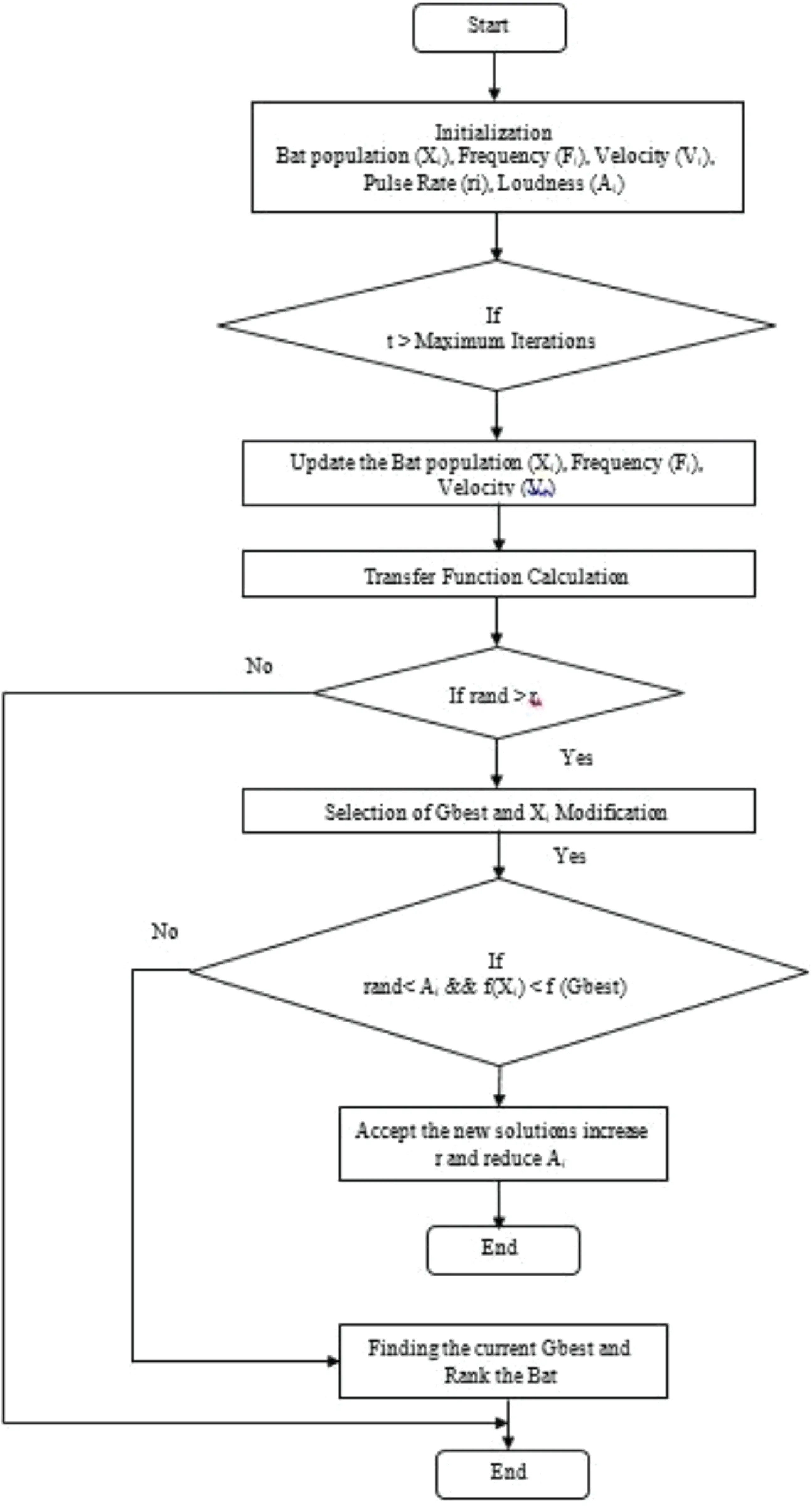Figure 2: Flow chart depicted for the bat algorithm

Each bat motion is associated with the velocity vit and initial distance xit with the ‘n’ number of iterations in a dimensional space or search space. The best bat of all the bats must be chosen based on the three rules mentioned above. The updated velocity vit and initial distance xit using the three rules are given below

Fi=fmin+(fmaxfmin)(7)

xit=xit1+vit(8)

where β € (0, 1) fmin is the minimum frequency = 0 and fmax is the maximum frequency which basically depends on the problem statement. The frequency between fmin and fmax is initially assigned to each bat. As a result, bat calculation can be thought of as a frequency tuning calculation to provide a fair mix of investigation and exploitation. The emission rates and loudness give a mechanism to programmed control and auto-zooming into the district with promising solutions.

To get good solution, it is fundamental for the variety of the loudness and the pulse emission. Since the loudness normally decreases once a bat has identified its prey, while the rate of pulse emission expands, the loudness can be picked as any estimation of accommodation, among Amin and Amax, accepting Amin = 0 implies that a bat has quite recently discovered the prey and briefly quit transmitting any stable.

4  Proposed Framework

4.1 System Overview

The proposed framework consists of four tier mechanism.

a)   Modelling the New Bat evolutionary Pruned Convolutional Layers

b)   Integrated with the Boosted LSTM Networks

c)   Implementation of Hybrid Algorithms on the FPGA with the Distributed Filtering Sections

d)   Adopting the Hardware -Software Co-design for an effective integration of the complete architecture. These frameworks

The CASIA video datasets are taken as the inputs to analysis of the proposed architecture. Initially the videos are converted into frames in terms of fixed point numeric values and features are extracted by the Bat pruned Convolutional Layers. These features are used to train the proposed boosted LSTM networks for further classification. The proposed architecture is shown in Fig. 3.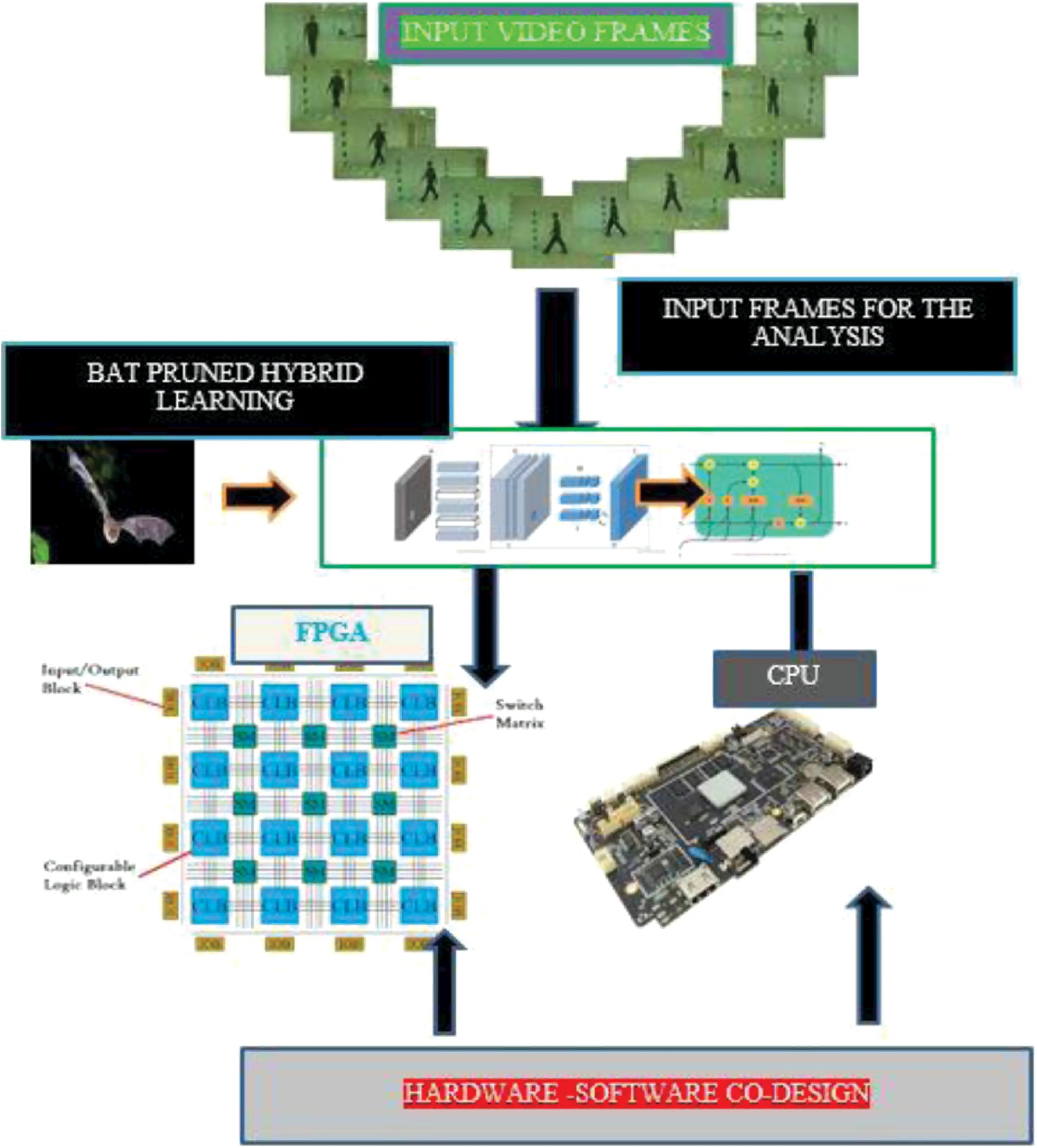Figure 3: Proposed architecture for the implementation of bpec-net frameworks

4.2 Bat Evolutionary Pruned Convolutional Networks

This section discusses about the details about each step involved in the proposed pruning methodology incorporated in convolutional layers. Even though the convolutional layers consist of three layers, pruning is incorporated in the filter layers of the proposed network. The proposed work uses the Adaptive Bat evolutionary algorithm for the filter pruning. Its goal is to remove the filters and to determine the impact of filter elimination on the loss function. The importance values that result can be used to prioritize the filters during the pruning process. The Bat algorithm is used to remove the filters with adaptive threshold fitness function given as follows

FitnessPruning<(L(D, f(i)L(D, f(j))(9)

where L(D, f(i) )is the Loss function after pruning by the bat algorithms, L(D, f(j)) is the loss function before the model perturbation. The input filter layers in accordance to the datasets are considered as the Bat populations and parameters used for pruning the filter layers are presented in Tab. 1.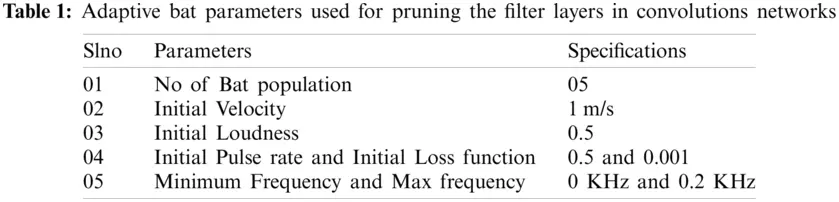The complete working mechanism of the proposed pruning mechanism is depicted in Fig. 4 and the Pseudo code is given below.

Sl. no. Pseudo Code for the Proposed Pruning Algorithm

01             Inputs: No of Filter layers = F(i)

02             Outputs: Pruned Output Layers = F(o)

03             Initial Bats = F(i)

04             Initialize the Loundness = 0.9

05             Initialize the Velocity = 1 m/s

06             While t = 1 to max_iteration

07             Loop1:     Pruned the Filter layers using adaptive thresholds

08                        Calculate the Loss function

09                       Calculate the Fitness function using the Equation (9)

10             If fitness_function < threshold (Again suing the Equation (9))

11                       Fix the Pruned Filter layer

12             Else

13                        Adjust the loudness, Velocity, Pulse rate and Frequency

14                           Go to Loop1

15              End

16             EndFigure 4: Hardware software co-design architecture for the proposed framework

The number of bats in the population, the maximum number of iterations, the upper bound, the lower bound, the dimension, the loudness, the pulse rate, and the maximum and minimum frequency range are all initialized as shown in Tab. 1. The number of filters and the number of neurons in the hidden layers of the convolutional layers are the parameters in CNN that must be pruned using the Bat algorithm.

From 1 to 50, the upper and lower bounds for the number of filters in the convolution layer and the number of neurons in the hidden layer are chosen at random.

4.3 Filter Tuning

The model structure is slightly weakened after removing the unnecessary filter layers, and its accuracy suffers as a result. To recover the accuracy, retraining the model is needed using the input video datasets. Since the important filter layers are retained, training accuracy can be retained in few epochs. But still, above methodology is not sufficient in handling the input video sequences because of its larger dimensions. Hence the proposed network replaces the traditional fully connected neural networks with the high performance boosted long short term memory for the training which retains the higher accuracy of prediction with the less number of filter layers. The training mechanism of the proposed LSTM is discussed as follows.

By combining LSTM networks and Ada-Boost Learning algorithms in this method, hybrid ensemble learning algorithms are developed. Ada-Boost algorithm, which strengthens weak classifiers. Normally, the Ada-boost algorithm improves the week classifiers by changing the classifier's weights. Until a high level of classification/prediction accuracy is achieved, Since the pruning can reduce the accuracy of training model, proposed Hybrid Models ensembles the Ada-Boost with the LSTM to handle the video sequences effectively with an increased prediction accuracy.

After the obtaining the features from pruned layers, the LSTM network is trained using Dk(i) which represents features obtained from the pruned filter layers of the convolutional networks Initially, D(i) are set equally, D1(i) = 1/n where n is the training samples. Then the network computes the weak LSTM predictor for the first iteration by using the mathematical Eq. (6). The modified output cell is given by

TC=kt TC~+TfTt1(10)

The mathematical expression identifies the threshold error function, which is used to find the boosting outputs.

Uk=(TactualTk)(11)

In addition, the network parameter has been calculated using the expression given by

αk=0.5{ln(1ek)/ek}(12)

When error is zero and the mathematical expression is given, ek is calculated for each iteration and the final ensembled boosted output is calculated.

F(k)  =k=0n1/αk{αk.Uk}(13)

The complete pseudo code for the proposed ensemble boosted LSTM is given below

Pseudo Code for the Proposed Boosted LSTM Predictor

1         Inputs Samples Training Sets {xi, yi}where x={x1, x2, x3, x5…….xn} where

n=Number of input video samples and yi €(1, 2, 3) where yi is multi-class label

associate with x

2         Initialize D(k)=n // features obtained from the Pruned Layers

3         For k = 1, 2, 3,…………….K

4               Train the LSTM classifier using the the distribution Dk

5                Get the hypothesis with error function with respective to Dk

6                     Error function is calculated at each stage which is then weighted Dk

7                         Choose αk = 0.5 {ln(1- ek)/ek}-network parameter calculation

8         Update the Dk+1 (i)// Ada Boost Mechanism

9            Calculate the error function and repeat the step 4

10        If error is less than ek

11                 Then Ensemble all the outputs H(x) = H(x)= sign(∑αk Tk)/ αk

12        Else

13        Go to step 4

14        End

15        End

By adopting the boosted LSTM with the pruned convolution layers, the high prediction accuracy has been maintained.

5  Experimental Setup

This section details about the FPGA implementation of the proposed pruned learning models. To overcome the computational overhead, the paper uses the hardware -software co-design which employs the pruned convolutional layers on FPGA and the boosted learning in the general central processing unit. Fig. 4 presents the complete cycle of implementing the proposed architecture.

For proposed Pruned Models, input videos are converted into matrix format using the matrix convertors and then stored in DRAM (On-chip RAM) for further processing. The block signal enabled distributed arithmetic architectures (BDA) are employed to implement the pruned weights of the proposed learning model. The filter co-efficient and input frame matric are then multiplied and accumulated by the BDA architecture followed by the memory read operations to other locations. The usage of the BDA mechanism incorporates the usage of registers (LUT) instead of the memories. Thus, proposed convolution layers eliminate the need for memory for storing filter coefficients Since the multiplication output is dependent on the number of bits used to represent data, this requires total B clock cycles for input of B bits.

All the computations using BDA architecture are conducted in the pipelined schemes for the fast processing and employs the parallel adders with a depth of log2(N) where n is the number of sample. Furthermore, array partitioning is done in the buffers’ corresponding dimensions to maximize bandwidth. In addition, for latency storage, double buffering storage is used. The pruned filter coefficients are then serially interfaced with the CPU, which operates the other layers, and then the boosted LSTM training mechanism is used to further classify activities.

6  Results and Discussion

This section discusses Results and discussion for the different datasets used for the experimentation, hardware specification, statistical analysis and resource utilizations with computational analysis.

6.1 Data Set Description

CASIA -B Datasets

CASIA database (http://www.cbsr.ia.ac.cn) is a standard data used in vision-based applications. It comprises of four distinct datasetsA, B, C, and D datasets represented in Tab. 2.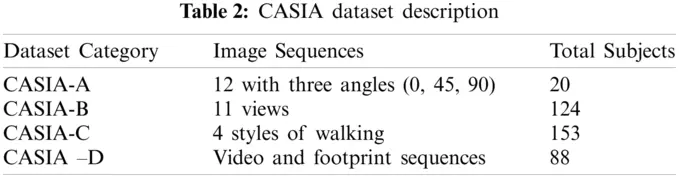6.2 Hardware Specification

As discussed earlier, ARTIX-7 FPGA is used for the implementing the pruned layers of the convolutional layers with the specification mentioned in Tab. 3 and the other learning Models are implemented in I7 CPU, 16GB RAM, 2TB HDD. The models in the CPU are developed by the Python 3.8 with Tensorflow API mechanism. The specification of Xilinx hardware is used for experimentation is presented in Tab. 3.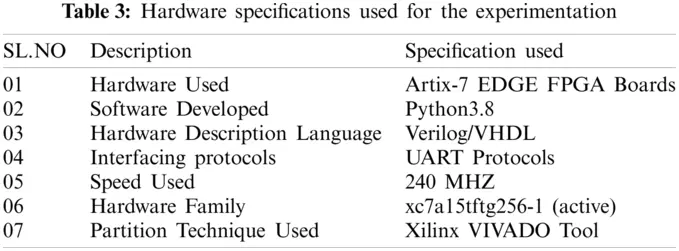6.3 Results and Discussion

Statistical Analysis

Using the proposed architecture, in order to test and validate the proposed intrusion detection method, we have calculated the different parameters such as Accuracy, Sensitivity, Selectivity, Specificity and evaluate the performance with the model without pruning techniques such as 3DCNN, CNN+LSTM, CNN. For each datasets, 70% has been taken as the training data and 30% as the testing data. The performance metrics estimation formulas are given below

Accuracy=DRTNI×100(11)

Sensitivity=TPTP+TN×100(12)

Specificity=TNTP+TN×100(13)

where TP and TN Represents True Positive and True Negative values and DR & TNI Represents Number of Detected Results and Total number of Iterations

Tab. 4 presents the relative results between the proposed algorithms with the unpruned CNN+LSTM hybrid learning models using the different datasets. The table infers that comparative analysis of the algorithms using the CASIA -A dataset for recognizing the human at 90 degrees and it is found the prediction accuracy of human recognition remains to be 97.5% for the both unpruned and pruned algorithms. For CASIA -B datasets prediction accuracy of human recognition remains to be 97.5% for the both unpruned and pruned algorithms. For CASIA-C and CASIA-D datasets the proposed algorithm and unpruned algorithm has produced the same prediction accuracy of 97.2% and 96.5% respectively. Hence, it is observed that the proposed hybrid model with the combination of boosted training model and pruned convolutional layers has produced the good accuracies for all datasets as same as unpruned hybrid model which can be suitable for the resource constraint hardware. To prove the efficiency of the proposed models, we have calculated the remaining parameters such as sensitivity and specificity whose analysis are depicted in Tab. 4.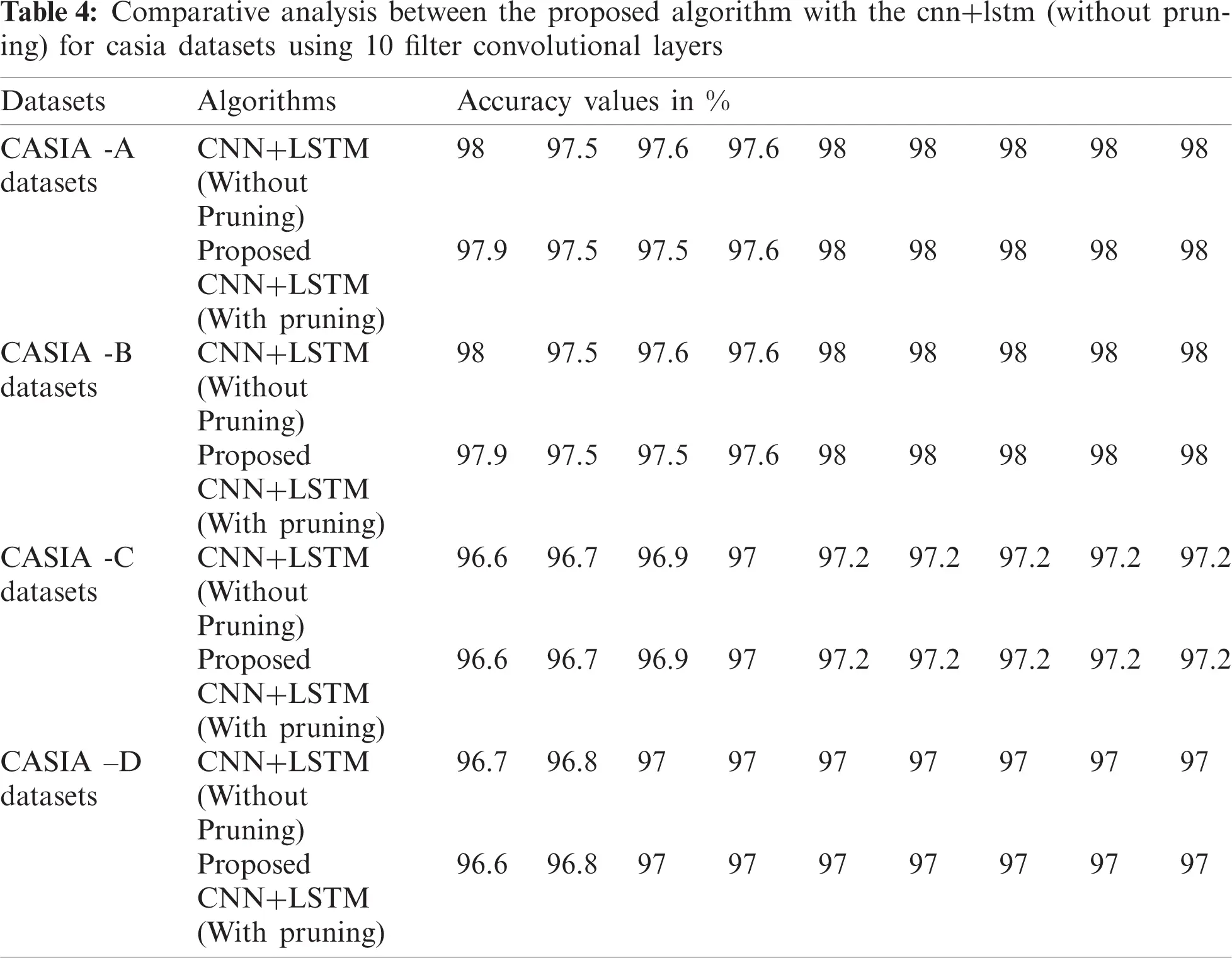Tab. 5 presents the sensitivity, specificity analysis between the proposed hybrid models with the unpruned hybrid model. It is found that sensitivity and specificity for the both algorithms are found to be 96.5%, 97.5% for CASIA-A and CASIA-B datasets, 97.0%, 96.5% for CASIA-C datasets, 96.0%, 96%, 5% respectively. In this analysis, it is observed that integration of the boosted training models along with the pruned layers constitutes the high accuracies as par with the other hybrid model.6.4 Design Space Exploration

Resource utilization

Since the proposed framework incorporates the hardware -software code sign, we have calculated the resources only for the pruned convolutional filter layers. The model architecture obtained for the proposed algorithms using different datasets are presented in the Tab. 6.Because of the limited resources on FPGA, the proposed blocked distributed arithmetic structure is required for FPGA computation, and the convolutional size parameters must be carefully chosen for efficient resource utilization. When it comes to memory and computation, the most important tools are BRAM (Block RAM) and DSP bricks. The ARTIX-7 FPGA architecture is used for the testing and implementation. Tab. 7 represents the resource utilization of the proposed pruned algorithm in ARTIX-7 FPGA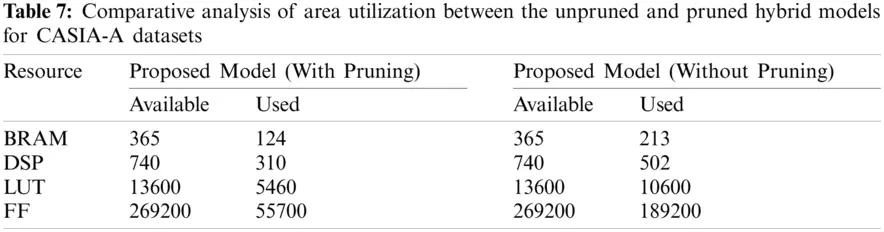The area utilization of the proposed model has been compared with the unpruned hybrid models and analysis are presented in the Tabs. 7--10. It is found that the Block Distributed Arithmetic architecture, which uses the principle of LUT storage instead of memory, has been used for implementing the pruned model utilizes only 40%-45% areas for predicting the different video datasets but the unpruned models consumed nearly 70% of its area in the FPGA. In addition, the reduction of the nodes in the filter layers and an effective block DA architecture has exhibited the effective memory utilization. Tab. 10 illustrates the efficiency of the proposed system validation process with its comparison results.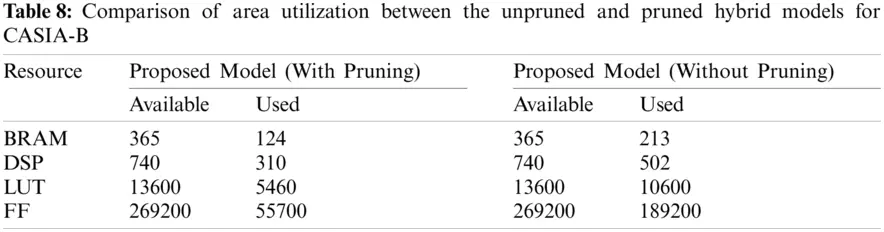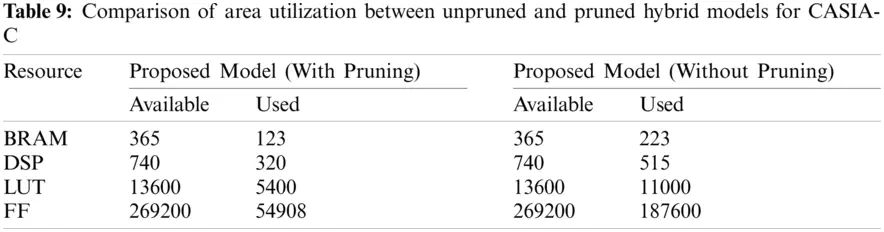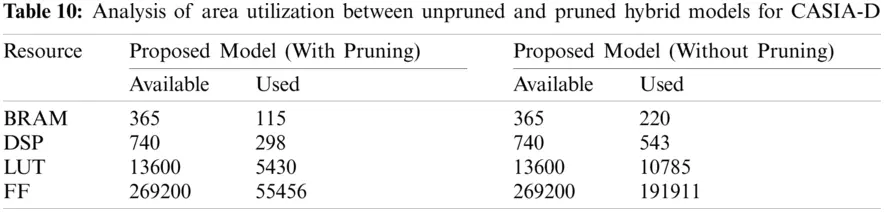Tab. 11 presents the comparative analysis between the different models used for the handling the different videos. It is found proposed models consumes 50% power less than CNN -LSTM model, 45% less than 3DCNN and 40% less than CNN Pruner models. The adoption of pruning in the filter layers and incorporation of hardware software codesign in the proposed model has considerably reduced the power consumption when associated with traditional models. Also the proposed model consumes only 40% of the total area which is 50% less than CNN-LSTM, 30% less than 3DCNN-pruned, 15% less than CNN –pruner models. The incorporation of LUT based Block DA architecture along with the array partition has consumed the reduced area and incorporation of double buffering mechanism has made the proposed model with 60% less delay than CNN-LSTM, 50% less than 3DCNN and 35% less than CNN-pruner models. From the above investigations, it is found that the proposed model finds its best suitability for handling the video sequences and established the good tradeoff between the prediction accuracy and computational resources.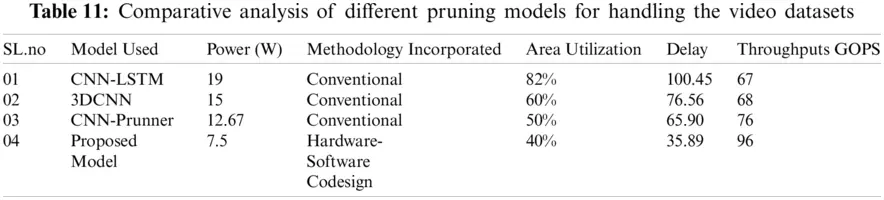7  Conclusion

In this work, evolutionary Bat is used for pruning filter layers of the convolutional neural network. To establish the good tradeoff between the pruning efficiency and prediction accuracy, the proposed model has incorporated the boosted LSTM along with the Bat pruned convolutional layer. To reduce the computational overhead, the proposed methodology incorporates the hardware-software codesign mechanism for the better implementation of the proposed models. Moreover, pruned filter weights are implemented using the novel Block Distributed Arithmetic (BDA) architectures in which the traditional memories are replaced with the LUTs, leads to the reduction of area utilization. The extensive experimentations are carried out using CASIA datasets and associated with traditional models. The prediction accuracy has been found to be 97% and consumes 50% power less than other existing algorithms such as CNN-Prunner, CNN-LSTM and 3DCNN. Moreover, the algorithms utilize only 40% of the resources compared to the 80% of the CNN-LSTM, 70% of 3DCNN and 65% of CNN prunner. From the above investigation, it is clear that the proposed pruned models have established the good tradeoff between the prediction accuracy and pruning efficiency. In future, more hybrid optimization algorithms can be applied to prune the filter layers and these techniques be extended to the other layers of Hybrid Convolutional Neural Networks.

Funding Statement: The authors received no specific funding for this study.

Conflicts of Interest: The authors declare that they have no conflicts of interest to report regarding the present study.

## References

1. J. Frankle and M. Carbin, “The lottery ticket hypothesis: finding sparse, trainable neural networks,” in Proc. 7th Int. Conf. on Learning Representations, New Orleans, LA, USA, 2019.
2. A. Krizhevsky, I. Sutskever and G. E. Hinton, “Imagenet classification with deep convolutional neural networks,” Communications of the ACM, vol. 60, no. 6, pp. 84–90, 2017.
3. K. Simonyan and A. Zisserman, “Very deep convolutional networks for large-scale image recognition,” in Proc. 3rd International Conf. on Learning Representations, San Diego, CA, USA, 2015.
4. D. Williams and G. Hinton, “Learning representations by back-propagating errors,” Nature, vol. 323, no. 6088, pp. 533–538, 1986.
5. S. Kirkpatrick, C. D. Gelatt and M. P. Vecchi, “Optimization by simulated annealing,” Science, vol. 220, no. 4598, pp. 671–680, 1983.
6. F. Glover, “Future paths for integer programming and links to artificial intelligence,” Computers & Operations Research, vol. 13, no. 5, pp. 533–549, 198
7. X. S. Yang, “A new metaheuristic bat-inspired algorithm,” in Proc. Nature Inspired Cooperative Strategies for Optimization (NICSO 2010Springer, Berlin, Heidelberg, pp. 65–74, 2010.
8. C. Xu, Z. Peng, X. Hu, W. Zhang, L. Chen et al., “FPGA-Based low-visibility enhancement accelerator for video sequence by adaptive histogram equalization with dynamic clip-threshold,” IEEE Transactions on Circuits and Systems–I: Regular Papers, vol. 67, no. 11, pp. 3954–3964, 2020.
9. G. Chen, Y. Ling, T. He, H. Meng, S. He et al., “Stereo engine: An FPGA-based accelerator for real-time high-quality stereo estimation with binary neural network,” IEEE Transactions on Computer-Aided Design of Integrated Circuits and Systems, vol. 39, no. 11, pp. 4179–4190, 2020.
10. Y. Kim, J. S. Choi, J. Lee and M. Kim, “A CNN-based multi-scale super-resolution architecture on FPGA for 4 K/8 K UHD applications,” in Proc. MMM 2020: 26th Int. Conf. on Multimedia Modelling, Daejeon, Korea, pp. 739–744, 2020.
11. W. Yang, Y. Gao and F. Zhai, “Simulation of sports action picture recognition based on FPGA and convolutional neural network,” Microprocessors and Microsystems, vol. 80, pp. 103593, 2021.
12. K. Cosmas and A. Kenichi, “Utilization of FPGA for onboard inference of landmark localization in CNN-based spacecraft pose estimation,” MDPI Journals-Aerospace, vol. 7, no. 11, pp. 159, 2020.
13. Z. Han, J. Jiang, L. Qiao, Y. Dou, J. Xu et al., “Accelerating event detection with DGCNN and FPGAs,” MDPI,” Electronics, vol. 9, no. 10, pp. 1666, 2020.
14. Y. H. Yu, Y. S. Ting, N. Kwok and N. M. Mayer, “High-speed gaze detection using a single FPGA for driver assistance systems,” Journal of Real-Time Image Processing, vol. 18, pp. 681–690, 2021.
15. X. Zhang, A. Ramachandran, C. Zhuge, D. He, W. Zuo et al., “Machine learning on FPGAs to face the IoT revolution,” in Proc. 2017 IEEE/ACM Int. Conf. on Computer-Aided Design (ICCADIrvine, CA, USA, pp. 894–901, 2017.
16. T. G. Nguyen, T. V. Phan, D. T. Hoang, T. N. Nguyen and C. So-In, “Efficient SDN-based traffic monitoring in IoT networks with double deep Q-network,” in Proc. Int. Conf. on Computational Data and Social Networks, Springer, Cham, pp. 26–38, 2020.This work is licensed under a Creative Commons Attribution 4.0 International License, which permits unrestricted use, distribution, and reproduction in any medium, provided the original work is properly cited.